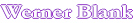# Home                              SOLUBILITY PARAMETER CALCULATION

This program calculates the solubility parameter and Tg of  an organic compound, oligomer or polymer from its individual components. This program works reasonably well for polyester, polyether and polyurethanes. For Tg calculations of acrylic or vinyl polymers the Fox equation is a more suitable program. The program uses additive cohesive energy calculation according to Feddors. For oligomers this program can also calculate the viscosity from Tg estimates according to  Kreibich &.Batzer . The method of Alemain is used to calculate the Tg of low molecular weight oligomers. The constant K in this equation can vary from 3x104 to 6x104 and will have to be determined. For oligomers with similar structure the same constant K can be used.  The WLF equation according to Nielsen is used to calculate the viscosity from Tg.
Limitations. The estimate of the Tg of an oligomer requires certain assumptions about the polymer structure. This equation assumes that the polymer is has random structure and is not crystalline. Longer side (>C9) chains in polymer can create side chain crystallinity which will effect the actual viscosity vs. calculated values. Selection of a the right K value for a polymer can compensate from some of these errors in oligomers. The viscosity is calculated at temperature of 25¯C. For polymers the number of groups entered reflects the number of groups in the polymer or oligomer to be calculated.
Enter Data (Total Number of Groups in the Polymer Chain has to be entered)
ATOM OR GROUP   Number of groups
-CH3
-CH2-
-CH<
>C<
H2C=
-CH=
>C=
H>C=
>C=
PHENYL
PHENYLENE (o,m,p)
PHENYL (TRI SUBSTUTED)
PHENYL (TETRA SUBSTUTED)
PHENYL (PENTA SUBSTUTED)
PHENYL (HEXA SUBSTUTED)
RING CLOSURE. =>5 ATOMS
RING CLOSURE. 3,4 ATOMS
CONJUGATION IN RING
-COOH
-O>C=O ESTER
>C=O KETONE
-CH=O ALDEHYDE
-O-C=O-C=O-O-  OXALATE
-C=O-O-C=O- ANHYDRIDE
-C=ONH2        AMIDE primary
-C=ONH-       AMIDE secondary
-NHC=O-O-   URETHANE
-C=ON<       AMIDE tertiary
O=CH-NH-
-NH2               AMINE primary
-NH-            AMINE secondary
-N<             AMINE tertiary
-N=
-CN             NITRILE
-NO2             NITRO aliphatic
-NO2          NITRO aromatic
-N=C=O        ISOCYANATE
-O-             ETHER
-OH HYDROXYL
-SH MERCAPTAN
-S- SULFIDE
ATOMS BETWEEN X-LINK  Will be used in future program expansions
Results
Solubility parameter, cal§cm-3/2
Molecular weight
Density g/cm3
Density lbs/gal
K = 10000 x Universal constant between 3 to 6
Tg compound, oligomer
Viscosity, log  (poise)
Viscosity, poise
Viscosity, cps

 Following Calculations are used: Cohesive energy calculations according to : R. F. Feddors., Poly. Eng. Sci. Vol. 14, No.2,147 (1974) Tg calculations according to: U. T. Kreibich, H.Batzer Ang. Macrom. Chem., 83, No.1281 (1979) 57 WLF equation according to: L. E. Nielsen, Polymer and Rheology, Marcel Dekker 1977, pp 33, 74, 133 Tg as a function of MW: J. V. Alemain J. Poly. Sci. Pol. Chem. Ed. 18 (1980) 256

Automatic recalculation

 Last edited on: October 20, 2010 CopyrightÛ, Design, Layout and Technical Content by: